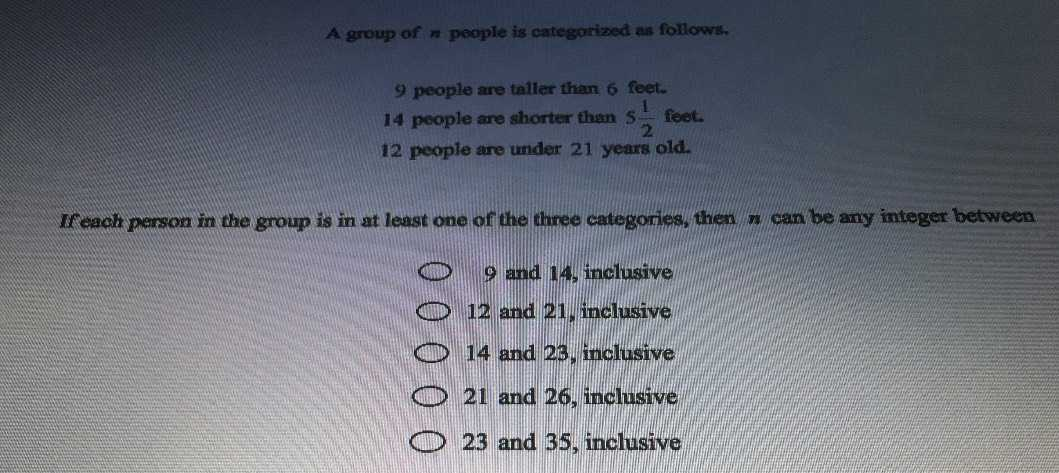### ¿Todavía tienes preguntas de matemáticas?

Pregunte a nuestros tutores expertos
Algebra
PreguntaA group of $$n$$ people is oategorized an follows,

$$9$$ people are taller than $$6$$ feet

$$14$$ poople are shorter then $$5 \frac { 1 } { 2 }$$ feet

$$12$$ people are under $$21$$ years old

If each person in the group is in at least one of the three categories, then n cam be any integer between

$$9$$ and $$4$$ , inclusive

$$( 12$$ and $$21$$ , incinasive

$$( 14$$ and $$23$$ , inclusine

$$( 21$$ and $$26$$ , inclusive

$$( 23$$ and $$35$$ , inclusive